NEET  >  NCERT Exemplar: Solid State

# NCERT Exemplar: Solid State - Chemistry Class 12 - NEET

MULTIPLE CHOICE QUESTIONS - I

Q.1. Which of the following conditions favours the existence of a substance in the solid state?
(i) High temperature
(ii) Low temperature
(iii) High thermal energy
(iv) Weak cohesive forces

Ans. (ii)
Solution.
At low temperature substance exists in solid state due to decrease in molecular motion which leads to strong cohesive forces i.e., forces which hold the constituent particles together.

Q.2. Which of the following is not a characteristic of a crystalline solid?
(i) Definite and characteristic heat of fusion.
(ii) Isotropic nature.
(iii) A regular periodically repeated pattern of arrangement of constituent particles in the entire crystal.
(iv) A true solid

Ans. (ii)
Solution.

Anisotropy: Crystalline solids are anisotropic in nature, that is some of their physical properties like electrical resistance or refractive index show different values when measured along different directions in the same crystal. This arises from different arrangement of particles in different directions arrangement of particles along different directions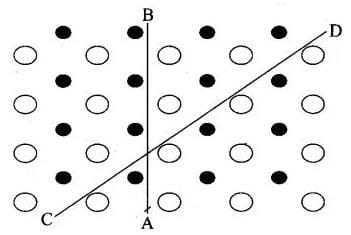Anisotropy in crystal is due to different arrangement of particles along different directions.

Isotropy: In case of amorphous substances, properties such as electrical conductivity, refractive index, thermal expansion, etc. are identical in all directions just as in case of gases or liquids. This property is called isotropy and the substances showing this property are called isotropic.

Q.3. Which of the following is an amorphous solid?
(i) Graphite (C)
(ii) Quartz glass (SiO2)
(iii) Chrome alum
(iv) Silicon carbide (SiC)
Ans. (ii)
Solution.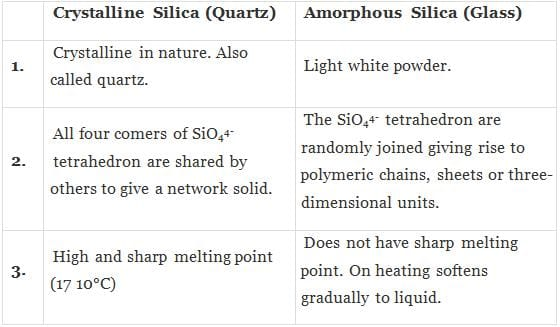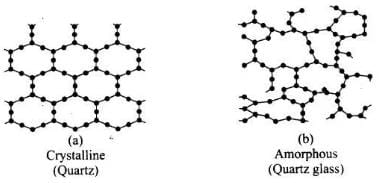Q.4. Which of the following arrangements shows schematic alignment of magnetic moments of antiferromagnetic substances?
(i)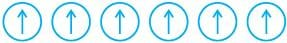(ii)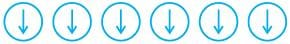(iii)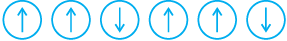(iv)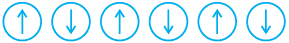Ans. (iv)
Solution.

The substances which have domain structure are oppositely oriented and cancel each other's magnetic field are known as antiferromagnetic substances.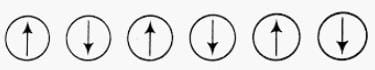Schematic arrangement of antiferromagnetic substance

Q.5. Which of the following is true about the value of refractive index of quartz glass?
(i) Same in all directions
(ii) Different in different directions
(iii) Cannot be measured
(iv) Always zero
Ans. (i)
Solution.

Since quartz glass is an amorphous solid having short range order of constitutents. Hence, value of refractive index is same in all directions, can be measured and not be equal to zero always.

Q.6. Which of the following statement is not true about amorphous solids?
(i) On heating they may become crystalline at certain temperature.
(ii) They may become crystalline on keeping for long time.
(iii) Amorphous solids can be moulded by heating.
(iv) They are anisotropic in nature.
Ans. (iv)
Solution.

Amorphous solids are isotropic in nature because it has no long range order and any physical property will be same in all directions. On the other hand, anisotropic nature is a characteristic feature of crystalline solid.

Q.7. The sharp melting point of crystalline solids is due to ___________.
(i) A regular arrangement of constituent particles observed over a short distance in the crystal lattice.
(ii) A regular arrangement of constituent particles observed over a long distance in the crystal lattice.
(iii) Same arrangement of constituent particles in different directions.
(iv) Different arrangement of constituent particles in different directions.
Ans. (ii)
Solution.

A solid is said to be crystalline if the various constituent structural units (atoms, ions or molecules) of which the solid is made, are arranged in a definite geometrical pattern within the solid.
The type of forces in crystalline solids are of long range order due to which they have sharp melting point.

Q.8. Iodine molecules are held in the crystals lattice by ____________.
(i) London forces
(ii) Dipole-dipole interactions
(iii) Covalent bonds
(iv) Coulombic forces
Ans. (i)
Solution.

Iodine molecules are a class of non-polar molecular solids in which constituent molecules are held together by weak London forces. These solids are soft and non-conductor of electricity.

Q.9. Which of the following is a network solid?
(i) SO2 (Solid)
(ii) I2
(iii) Diamond
(iv) H2O (Ice)
Ans. (iii)
Solution.

Diamond is a giant molecule in which constituent atoms are held together by covalent bond.
Hence, this is a network solid.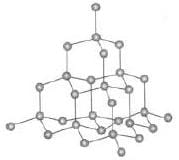Network structure of atom

Q.10. Which of the following solids is not an electrical conductor?
(A) Mg (s)
(B) TiO (s)
(C) I2(s)
(D) H2O (s)
(i) (A) only
(ii) (B) Only
(iii) (C) and (D)
(iv) (B), (C) and (D)
Ans. (iii)
Solution.

Iodine is a non-polar molecular solid in which iodine molecules are held together by London force or dispersion force. This is soft and non-conductor of electricity.
Water is a hydrogen bonded molecular solid in which H and 0 are held together by polar covalent bond and each water molecule held together by hydrogen bonding. Due to non-ionic nature, they are not electrical conductor.

Q.11. Which of the following is not the characteristic of ionic solids?
(i) Very low value of electrical conductivity in the molten state.
(ii) Brittle nature.
(iii) Very strong forces of interactions.
(iv) Anisotropic nature.
Ans. (i)
Solution.

Ionic solids easily dissociated into its ions in molten state and show high electrical conductivity. So, statement (i) is incorrect while ionic solids are anisotropic and brittle linked with very strong force of interactions.

Q.12. Graphite is a good conductor of electricity due to the presence of __________.
(i) Lone pair of electrons
(ii) Free valence electrons
(iii) Cations
(iv) Anions
Ans. (ii)
Solution.

Graphite is a good conductor of electricity due to presence of free valence electrons. In graphite, each carbon is sp2 hybridised having one free electron which makes graphite a good conductor of electricity.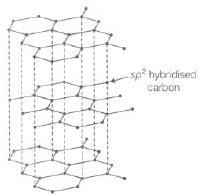Q.13. Which of the following oxides behaves as conductor or insulator depending upon temperature?
(i) TiO
(ii) SiO2
(iii) TiO3
(iv) MgO
Ans. (iii)
Solution.

Certain metal oxides like V02, VO, VO3 and TiO3 show metallic or insulating property depending upon temperature. As temperature varies metallic or insulating property varies. This is due to variation in energy gap between conduction band and valence band.

Q.14. Which of the following oxides shows electrical properties like metals?
(i) SiO2
(ii) MgO
(iii) SO2(s)
(iv) CrO2
Ans. (iv)
Solution.
CrO2, TiO and Re03 are some typical metal oxides which show electrical conductivity similar to metal. While SO2, MgO and SO2 are oxides of metal, semimetal and non-metal which do not show electrical properties.

Q.15. The lattice site in a pure crystal cannot be occupied by _________.
(i) Molecule
(ii) Ion
(iii) Electron
(iv) Atom
Ans. (iii)
Solution.

Pure crystals have constituents i.e., atoms or molecules or ions as lattice points which are arranged in fixed stoichiometric ratio. Electron can occupy the lattice site only when there is imperfection in solid and not in a pure crystal.
Hence, existence of free electrons are not possible, it is possible on in case of imperfection in solid.

Q.16. Graphite cannot be classified as __________.
(i) Conducting solid
(ii) Network solid
(iii) Covalent solid
(iv) Ionic solid

Ans. (iv)
Solution.

Constituent units of graphite are carbon atoms, held together by covalent bonding in 2D network structure. Thus, it is not an ionic solid.

Q.17. Cations are present in the interstitial sites in __________.
(i) Frenkel defect
(ii) Schottky defect
(iii) Vacancy defect
(iv) Metal deficiency defect
Ans. (i)
Solution.

When smaller ion (usually cation) is dislocated from its normal site in crystal and move to interstitial site is known as Frenkel defect as shown below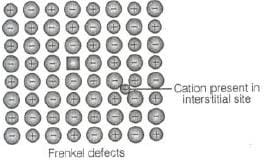Q.18. Schottky defect is observed in crystals when __________.
(i) Some cations move from their lattice site to interstitial sites.
(ii) Equal number of cations and anions are missing from the lattice.
(iii) Some lattice sites are occupied by electrons.
(iv) Some impurity is present in the lattice.

Ans. (ii)
Solution.

Schottky defect is observed in crystal when equal number of cations and anions are missing from the lattice.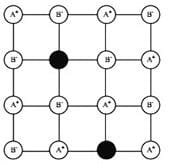Schottkey defect

When some cations move from their lattice site to interstitial site, this defect is known as Frenkel defect. When some impurity is present in crystal lattice it is known as impurity defect.
When lattice site is occupied by electron, this type of defect is known as metal excess defect.

Q.19. Which of the following is true about the charge acquired by p -type semiconductors?
(i) Positive
(ii) Neutral
(iii) Negative
(iv) Depends on concentration of p impurity
Ans. (ii)
Solution.

When group 13 elements are doped into the lattice of group 14 element, it creates a hole in amolecule but the molecule as a whole remains neutral. Thus, p -type semiconductor is neutral in nature.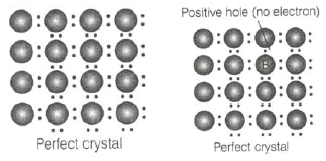Q.20. To get a n-type semiconductor from silicon, it should be doped with a substance with valence__________.
(i) 2
(ii) 1
(iii) 3
(iv) 5
Ans. (iv)
Solution.

Impurity of higher group is doped to get n-type semiconductor. Thus, silicon (valency = 4) should be doped with the element with valency equal to 5.

Q.21. The total number of tetrahedral voids in the face centred unit cell is __________.
(i) 6
(ii) 8
(iii) 10
(iv) 12
Ans. (ii)
Solution.

Fee unit cell contains 8 tetrahedral voids at centre of each 8 smaller cube of a unit cell as shown below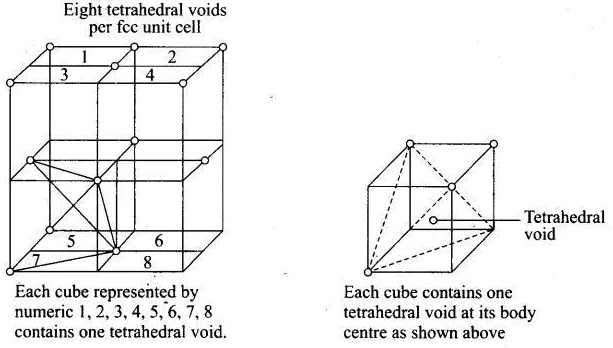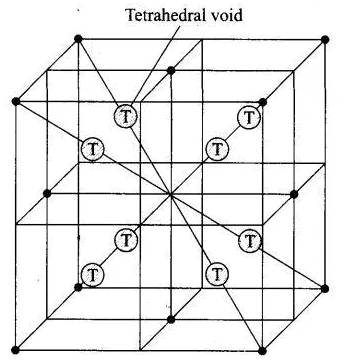Q.22. Which of the following point defects are shown by AgBr(s) crystals?
(A) Schottky defect
(B) Frenkel defect
(C) Metal excess defect
(D) Metal deficiency defect

(i) (A) and (B)
(ii) (C) and (D)
(iii) (A) and (C)
(iv) (B) and (D)

Ans. (i)
Solution.

AgBr shows both Schottky and Frenkel defects. In AgBr, both Ag+ and Br ions are absent from the lattice causing Schottky defect. However, Ag+ ions are mobile so they have a tendency to move aside the lattice and trapped in interstitial site, hence cause Frenkel defect.

Q.23. In which pair most efficient packing is present?
(i) hcp and bcc
(ii) hcp and ccp
(iii) bcc and ccp
(iv) bcc and simple cubic cell
Ans. (ii)
Solution.

Packing efficiency: It is the percentage of total filled space by particles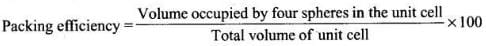Since, packing efficiency for hep or ccp is calculated to be 74% which is maximum among all type of crystals.

Q.24. The percentage of empty space in a body centred cubic arrangement is ________.
(i) 74
(ii) 68
(iii) 32
(iv) 26
Ans. (iii)
Solution.

Packing efficiency for bcc arrangement is 68% which represents total filled space in the unit cell. Hence, empty space in a body centered arrangement is 100 – 68 = 32%

Q.25. Which of the following statement is not true about the hexagonal close packing?
(i) The coordination number is 12.
(ii) It has 74% packing efficiency.
(iii) Tetrahedral voids of the second layer are covered by the spheres of the third layer.
(iv) In this arrangement spheres of the fourth layer are exactly aligned with those of the first layer.
Ans. (iv)
Solution.

Hexagonal close packing can be arranged by two layers
A and B one over another which can be diagrammatically represented as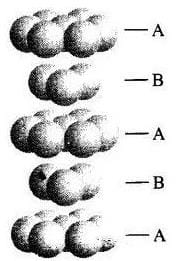Hexagonal Close Packing

Here, we can see easily that 1st layer and 4th layer are not exactly aligned. Thus, statement (d) is not correct while other statements (a), (b) and (c) are true.

Q.26. In which of the following structures coordination number for cations and anions in the packed structure will be same?
(i) Cl ion form fcc lattice and Na+ ions occupy all octahedral voids of the unit cell.
(ii) Ca2+ ions form fcc lattice and F – ions occupy all the eight tetrahedral voids of the unit cell.
(iii) O2– ions form fcc lattice and Na+ ions occupy all the eight tetrahedral voids of the unit cell.
(iv) S2– ions form fcc lattice and Zn2+ ions go into alternate tetrahedral voids of the unit cell.
Ans. (i)
Solution.

NaCl crystals have rock salt structure having fee lattice in which Cl ions are present at fee lattice points and face centre and Na+ occupies all the octahedral voids of given unit cell.
Where, coordination number of Na+ = 6
Coordination number of Cl= 6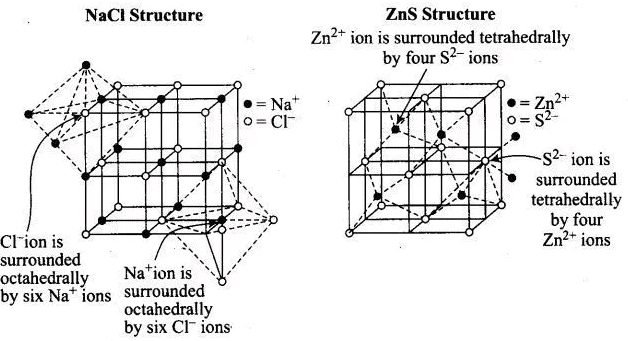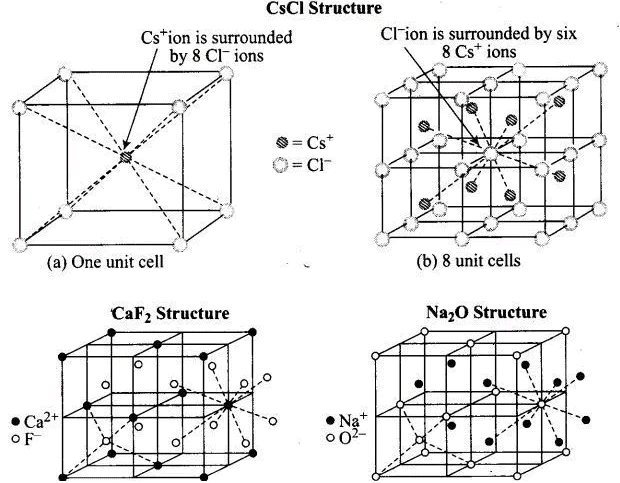Q.27. What is the coordination number in a square close packed structure in two dimensions?
(i) 2
(ii) 3
(iii) 4
(iv) 6
Ans. (iii)
Solution.

Coordination number in a square closed packed structure in two dimensions is equal to 4 is shown as: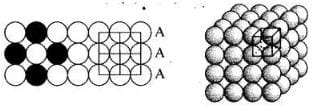Q.28. Which kind of defects are introduced by doping?
(i) Dislocation defect
(ii) Schottky defect
(iii) Frenkel defects
(iv) Electronic defects
Ans. (iv)
Solution.

When electron rich or electron deficient impurity is added to a perfect crystal, it introduces electronic defect in them.

Q.29. Silicon doped with electron-rich impurity forms  ________.
(i) p-type semiconductor
(ii) n-type semiconductor
(iii) intrinsic semiconductor
(iv) insulator
Ans. (ii)
Solution.

Silicon has four valence electrons. If it is doped with an electron rich impurity, the extra electron becomes delocalised and increases the conductivity. Since the increase in conductivity is due to negatively charged electron, hence it is called n-type semiconductor.

Q.30. Which of the following statements is not true?
(i) Paramagnetic substances are weakly attracted by magnetic field.
(ii) Ferromagnetic substances cannot be magnetized permanently.
(iii) The domains in antiferromagnetic substances are oppositely oriented with respect to each other.
(iv) Pairing of electrons cancels their magnetic moment in the diamagnetic substances.
Ans. (ii)
Solution.

Ferromagnetic species are strongly attracted in the magnetic field and can be permanently magnetised.
Hence, choice (ii) is the correct answer while other three choices are correct.

Q.31. Which of the following is not true about the ionic solids?
(i) Bigger ions form the close packed structure.
(ii) Smaller ions occupy either the tetrahedral or the octahedral voids depending upon their size.
(iii) Occupation of all the voids is not necessary.
(iv) The fraction of octahedral or tetrahedral voids occupied depends upon the radii of the ions occupying the voids.
Ans. (iv)
Solution.

The fraction of octahedral or tetrahedral voids occupied depends upon the radii of the ions present at the lattice points. As we know the radii of octahedral or tetrahedral void is related to radii of atoms (r) as Radius of octahedral void (R0) = 0.414 r.
Radius of tetrahedral void (R1) = 0.225 r  Where, r = radius of bigger atom involved.

Q.32. A ferromagnetic substance becomes a permanent magnet when it is placed in a magnetic field becuase ________.
(i) All the domains get oriented in the direction of magnetic field.
(ii) All the domains get oriented in the direction opposite to the direction of magnetic field.
(iii) Domains get oriented randomly.
(iv) Domains are not affected by magnetic field.

Ans. (i)
Solution.

Ferromagnetic solids can be permanently magnetised and then all the domains get oriented in the direction of applied magnetic field.

Q.33. The correct order of the packing efficiency in different types of unit cells is ________.
(i) fcc < bcc < simple cubic
(ii) fcc > bcc > simple cubic
(iii) fcc < bcc > simple cubic
(iv) bcc < fcc > simple cubic
Ans. (ii)
Solution.

Packing efficiency in different types of unit cells can be tabulated as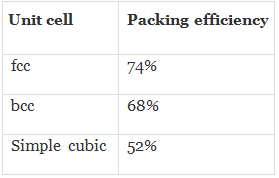Hence, correct order is fee (74%) > bee (68%) > simple cubic (52%).

Q.34. Which of the following defects is also known as dislocation defect?
(i) Frenkel defect
(ii) Schottky defect
(iii) Non-stoichiometric defect
(iv) Simple interstitial defect
Ans. (i)
Solution.

In Frenkel defect, some cations occupy interstitial site and hence it is also called dislocation defect.

Q.35. In the cubic close packing, the unit cell has ________.
(i) 4 tetrahedral voids each of which is shared by four adjacent unit cells.
(ii) 4 tetrahedral voids within the unit cell.
(iii) 8 tetrahedral voids each of the which is shared by four adjacent unit cells.
(iv) 8 tetrahedral voids within the unit cells.
Ans. (iv)
Solution.

In the cubic close packing the unit cell has 8 tetrahedral voids within it and are located at each eight smaller cube of the unit cell

Q.36. The edge lengths of the unit cells in terms of the radius of spheres constituting fcc, bcc and simple cubic unit cell are respectively________.
(i)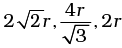(ii)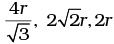(iii)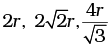(iv)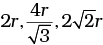Ans. (i)
Solution.

Note: Distance between two atoms is always measured from their centres
(i) If the crystal lattice consists of SCC, the atom which is present at the comers touch each other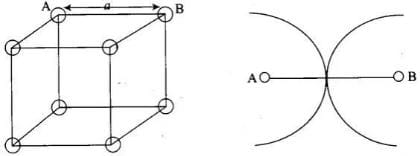(ii) In case of FCC, atom present at the comer and the centre of the face touch each other.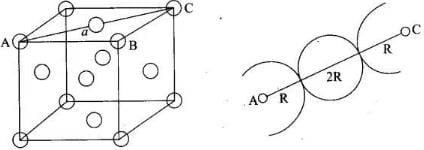(iii) In case of BCC atom present at the corner and center of the body touch each other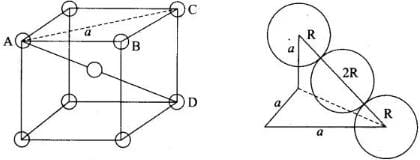Edge length for different types of unit cells can be tabulated as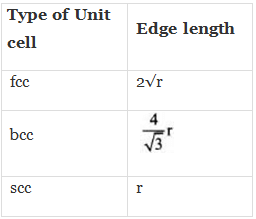Q.37. Which of the following represents correct order of conductivity in solids?
(i) κmetals >> κinsulators < κsemiconductors
(ii) κmetals<< κinsulators < κsemiconductors
(iii) κmetals < κsemiconductors > κinsulators = zero
(iv) κmetals < κsemiconductors > κinsulators ≠ zero
Ans. (i)
Solution.

Conductivity of metal, insulator and semiconductors can be represented in the term of k (Kappa) which depends upon energy gap between valence band and conduction band.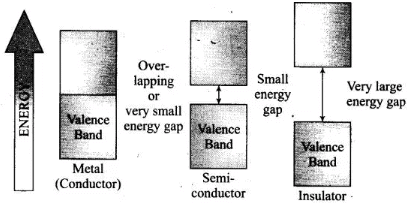Valence and Conductance bands in metals, semiconductors and insulators.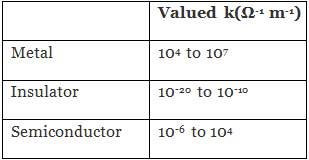MULTIPLE CHOICE QUESTIONS - II

Note : In the following questions two or more options may be correct.
Q.38. Which of the following is not true about the voids formed in 3 dimensional hexagonal close packed structure?
(i) A tetrahedral void is formed when a sphere of the second layer is present above triangular void in the first layer.
(ii) All the triangular voids are not covered by the spheres of the second layer.
(iii) Tetrahedral voids are formed when the triangular voids in the second layer lie above the triangular voids in the first layer and the triangular shapes of these voids do not overlap.
(iv) Octahedral voids are formed when the triangular voids in the second layer exactly overlap with similar voids in the first layer.
Ans. (iii, iv)
Solution.
Tetrahedral voids are formed when the triangular void in the second layer lie exactly above the triangular voids in the first layer and the triangular shape of these voids oppositely overlap.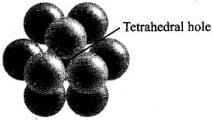Octahedral voids are formed when triangular void of second layer is not exactly overlap with similar void in first layer.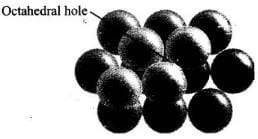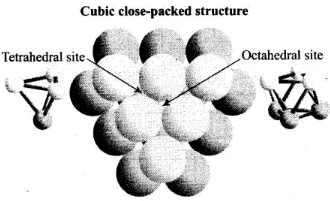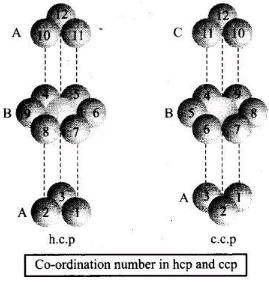Q.39. The value of magnetic moment is zero in the case of antiferromagnetic substances because the domains ________.
(i) Get oriented in the direction of the applied magnetic field.
(ii) Get oriented opposite to the direction of the applied magnetic field.
(iii) Are oppositely oriented with respect to each other without the application of magnetic field.
(iv) Cancel out each other’s magnetic moment.
Ans. (iii, iv)
Solution.

In the case of antiferromagnetic substances, the magnetic moment becomes zero because the domains are oppositely oriented with respect to each other without the application of magnetic field which cancel out each other.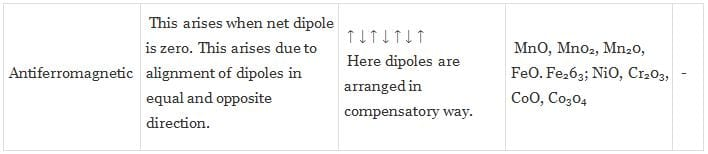Q.40. Which of the following statements are not true?
(i) Vacancy defect results in a decrease in the density of the substance.
(ii) Interstitial defects results in an increase in the density of the substance.
(iii) Impurity defect has no effect on the density of the substance.
(iv) Frankel defect results in an increase in the density of the substance.
Ans. (iii, iv)
Solution.

Statements (iii) and (iv) can be correctly written as (iii) Impurity defect
changes the density of substance as impurity has different than the ion present on perfect crystal e.g., When SrCl2 is added to the NaCl crystal, it causes impurity defect, (iv) Frenkel defect results neither decrease nor increase in density of substance.

Q.41. Which of the following statements are true about metals?
(i) Valence band overlaps with conduction band.
(ii) The gap between valence band and conduction band is negligible.
(iii) The gap between valence band and conduction band cannot be determined.
(iv) Valence band may remain partially filled.
Ans. (i, ii, iv)
Solution.

In metal, valence band overlap with conduction band. The gap between valence band and conduction band is negligible and valence band may remain partially filled.

Q.42. Under the influence of electric field, which of the following statements is true about the movement of electrons and holes in a p-type semi conductor?
(i) Electron will move towards the positively charged plate through electron holes.
(ii) Holes will appear to be moving towards the negatively charged plate.
(iii) Both electrons and holes appear to move towards the positively charged plate.
(iv) Movement of electrons is not related to the movement of holes.
Ans. (i, ii)
Solution.

In p-type semiconductor, the conductivity is due to existence of hole. When electric field is applied to p-type semiconductor hole starts moving towards negatively charged plate and electron towards positively charged plate.
Flow of holes in p-type semiconductors Hole .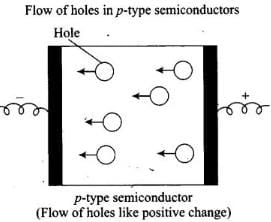Q.43. Which of the following statements are true about semiconductors?
(i) Silicon doped with electron rich impurity is a p-type semiconductor.
(ii) Silicon doped with an electron rich impurity is an n-type semiconductor.
(iii) Delocalised electrons increase the conductivity of doped silicon.
(iv) An electron vacancy increases the conductivity of n-type semiconductor.

Ans. (ii, iii)
Solution.

Silicon (valence electron – 4) doped with electron rich impurity is an n-type semiconductor due to extra electron and the delocalised electrons increase the conductivity of doped silicon.

Q.44. An excess of potassium ions makes KCl crystals appear violet or lilac in colour since ________.
(i) Some of the anionic sites are occupied by an unpaired electron.
(ii) Some of the anionic sites are occupied by a pair of electrons.
(iii) There are vacancies at some anionic sites.
(iv) F-centres are created which impart colour to the crystals.
Ans. (i, iv)
Solution.

When KC1 is heated in vapour of K, some of the Cl” leave their lattice site and create anion vacancies. This chloride ion wants to combine with K vapour to form potassium chloride. For doing so K atom loses electrons form K ions. This released electron diffuses into the crystal to get entrapped in the anion vacancy called F-centre. When visible light falls on the crystal, this entrapped electron gains energy, goes to the higher level when it comes back to the ground state, energy is released in the form of light.

Q.45. The number of tetrahedral voids per unit cell in NaCl crystal is ________.
(i) 4
(ii) 8
(iii) Twice the number of octahedral voids.
(iv) Four times the number of octahedral voids.
Ans. (ii, iii)
Solution.

NaCl has fee arrangement of CF ions. Thus,
Number of CF ions in packing per unit cell = 4
Number of tetrahedral voids = 2 x No. of particles present in close packing
= 2 × 4 = 8
Number of tetrahedral voids = 2 x No. of octahedral voids

Q.46. Amorphous solid can also be called ________.
(i) Pseudo solids
(ii) True solids
(iii) Super cooled liquids
(iv) Super cooled solids
Ans. (i, iii)
Solution.

Amorphous solid has short range order which has a tendency to flow very slowly. Hence, it is also known as pseudo solids or super cooled liquids. Glass panes fixed to windows or doors of old buildings are invariably observed to be thicker at bottom than at the top. These are examples of amorphous solids.

Q.47. A perfect crystal of silicon (Fig. 1.1) is doped with some elements as given in the options. Which of these options show n-type semiconductors?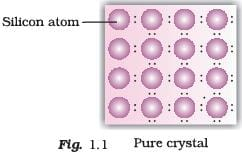(i)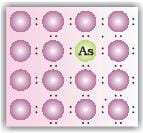(ii)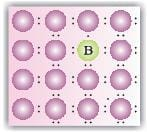(iii)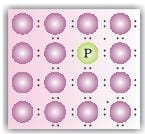(iv)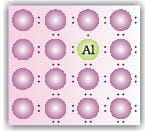Ans. (i, iii)
Solution.
When group 15 elements are doped into a perfect crystal, it leads to the formation of n-type semiconductor.
Here, in (a) as (group 15, period 3) is doped to perfect Si-crystal and in (c) as (group 15, period 2) is doped to perfect Si-crystal.

Q.48. Which of the following statements are correct?
(i) Ferrimagnetic substances lose ferrimagnetism on heating and become paramagnetic.
(ii) Ferrimagnetic substances do not lose ferrimagnetism on heating and remain ferrimagnetic.
(iii) Antiferromagnetic substances have domain structures similar to ferromagnetic substances and their magnetic moments are not cancelled by each other.
(iv) In ferromagnetic substances all the domains get oriented in the direction of magnetic field and remain as such even after removing magnetic field.

Ans. (i, iv)
Solution.

Ferrimagnetic substances lose ferrimagnetism on heating and become paramagnetic. In ferromagnetic substance, domains are aligned in parallel and antiparallel direction in unequal numbers.
In ferromagnetic substances, all the domains get oriented in the direction of magnetic field and remain as such even after removing magnetic field.

Q.49. Which of the following features are not shown by quartz glass?
(i) This is a crystalline solid.
(ii) Refractive index is same in all the directions.
(iii) This has definite heat of fusion.
(iv) This is also called super cooled liquid.

Ans. (i, iii)
Solution.

Quartz glass is an amorphous solid so it has not definite heat of fusion. This is due to short range order of molecule while quartz glass is also known as super cooled liquid and isotropic in nature.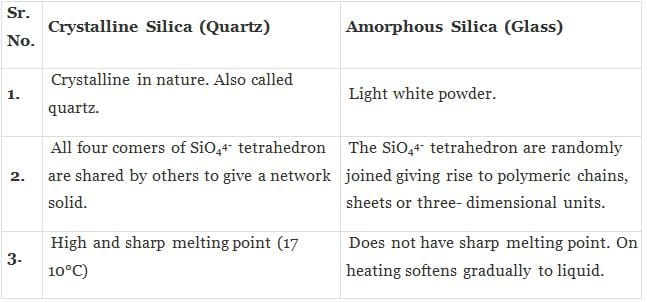Q.50. Which of the following cannot be regarded as molecular solid?
(i) SiC (Silicon carbide)
(ii) AlN
(iii) Diamond
(iv) I2
Ans. (i, ii, iii)
Solution.

SiC, AIN and diamond are examples of network solid as they have three dimensional structure while, I2 is a molecular solid, because such solid particles are held together by dipole-dipole interactions. SiC and AIN are interstitial solids.

Q.51. In which of the following arrangements octahedral voids are formed?
(i) hcp
(ii) bcc
(iii) simple cubic
(iv) fcc
Ans. (i, iv)
Solution.

In hcp and fee arrangement, octahedral voids are formed. In fcc, the octahedral voids are observed at edge and centre of cube while in bcc and simple cubic, no any octahedral voids are observed. In bcc, cubic voids formed.

Q.52. Frenkel defect is also known as ________.
(i) Stoichiometric defect
(ii) Dislocation defect
(iii) Impurity defect
(iv) Non-stoichometric defect
Ans. (i, ii)
Solution.

In Frenkel defect, dislocation of cations takes place and there is no change in stoichiometry of the crystal.

Q.53. Which of the following defects decrease the density?
(i) Interstitial defect
(ii) Vacancy defect
(iii) Frankel defect
(iv) Schottky defect
Ans. (ii, iv)
Solution.

Vacancy and Schottky defect which lead to decrease the density both are the types of a stoichiometric defect. In case of Frenkel defect and interstitial defect, there is no change in density of substance.

## Part- 2

Q.54. Why are liquids and gases categorised as fluids?
Ans. The liquids and gases have a property to flow i.e., the molecules of liquids and gases can easily move fast and tumble over one another freely. Because of their tendency to flow, these have been categorized as fluids.

Q.55. Why are solids incompressible?
Ans. The intemuclear distance between the constituent particles (atoms, molecules or ions) in solids are very less. On bringing them further closer, there will be large repulsive force between electron clouds of these particles. Therefore, solids cannot be compressed.

Q.56. Inspite of long range order in the arrangement of particles why are the crystals usually not perfect?
Ans. Crystals have long range in the arrangement of particles but usually the crystals are not perfect this is because when crystallisation occurs at a fast rate or moderate rate, the constituent particles may not get sufficient time to arrange themselves in a perfect order.

Q.57. Why does table salt, NaCl, some times appear yellow in colour?
Ans. The yellow colour of sodium chloride crystals is due to metal excess defect. In this defect, the unpaired electrons get trapped in anion vacancies. These sites are called F-centres. The‘yellow colour results by excitation of these electrons when they absorb energy from the visible light falling on the crystals.

Q.58. Why is FeO(s) not formed in stoichiometric composition?
Ans. Iron oxide (FeO) has rock salt structure.
In this case, O2 ions adopt on sites and Fe2+ ions should occupy non- stoichiometric. This is the ideal arrangement.
This oxide is always non-stoichiometric i.e., the composition of Fe2+ and O2 ions is not 1 : 1. It is 0.95 : 1
i.e. Fe0.95(Wustite)
This composition can be obtained if a small number of Fe2+ ions are replaced by two-thirds of Fe3+ ions in Oh sites.
Eventually there would be less amount of metal as compared to stoichiometric composition.

Q.59. Why does white ZnO(s) becomes yellow upon heating?
Ans. When ZnO is heated, it splits up to give Zn2+, electrons and colour because of the following reasons:
The excess Zn ions thus formed get entrapped in the interstitial site and electron in the neighborhood vacant interstitial sites. This electron is responsible for the colour and electrical conductivity in crystals.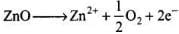• ZnO heated in Zn vapour — Zny0 (y > I) • The excess cations occupy interstitial voids • The electrons (2e-) released stay associated to the interstitial cation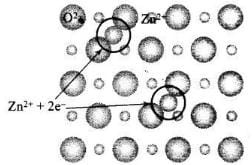Q.60. Why does the electrical conductivity of semiconductors increase with rise in temperature?
Ans. The energy gap between valence band and conduction band is small. At room temperature, they do not conduct electricity but when temperature is raised large number of electron from valence band get sufficient energy to jump to conduction band. This is known as thermodynamic conduction in intrinsic semiconductors. Thus, they become more conducting as the temperature increases.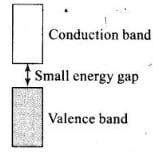Q.61. Explain why does conductivity of germanium crystals increase on doping with gallium.
Ans. p-type semiconductor: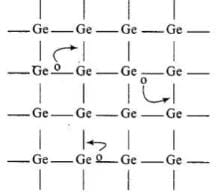• When Ge is doped with group 13 elements, for example, gallium, the structure of crystal lattice does not change.
• 3 valence electrons of gallium are used up in the normal covalent bond.
• For one dopant atom, one hole is created because the place where fourth electron is missing is called vacancy or hole and is responsible for conduction of germanium doped with gallium.

Electron from neighbouring atom comes and fills the hole, thereby creating a hole in its original position.Under the influence of electric field electrons move towards positively charged plates through these and conduct electricity. The holes appear to move towards negatively charged plates.

Q.62. In a compound, nitrogen atoms (N) make cubic close packed lattice and metal atoms (M) occupy one-third of the tetrahedral voids present. Determine the formula of the compound formed by M and N?
Ans. In ccp, no. of atoms per unit cell = 4
Thus, of tetrahedral voids = 2 x No. of atoms in ccp = 2 x 4 = 8
Only one-third of tetrahedral voids are occupied by metal M. No. of
No. of metal atoms, M = 1/ x 8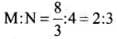Thus, formula is M2N3.

Q.63. Under which situations can an amorphous substance change to crystalline form?
Ans. An amorphous solid on heating at some temperature may become crystalline. Slow heating and cooling over a long period makes an amorphous solid acquires some crystalline character.

MATCHING TYPE

Note : In the following questions match the items given in Column I with the items given in Column II. In some questions more than one item of Column I and Column II may match.
Q.64. Match the defects given in Column I with the statements in given Column II.

 Column I Column II (i) Simple vacancy defect (a) shown by non-ionic solids and increases density of the solid. (ii) Simple interstitial defect (b) shown by ionic solids and decreases density of the solid. (iii) Frenkel defect (c) shown by non ionic solids and density of the solid decreases (iv) Schottky defect (d) shown by ionic solids and density of the solid remains the same.

Ans. (i) → (c); (ii) → (a); (iii) → (d); (iv) → (b)
Solution.
(i) When some of lattice sites are vacant in any non-ionic solid, the crystal is said to have vacancy defect and due to decrease in number of particles present in crystal lattice the density of crystal decreases.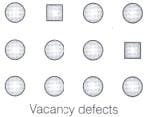(ii) Simple interstitial defect are shown by non-ionic solids in which constituent particles is displaced from its normal site to an interstitial site. Hence, density of solid increases.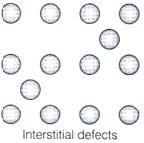(iii) Frenkel defect is shown by ionic solids in which smaller ions get dislocated from its normal site to its interstitial site which lead to decrease its density.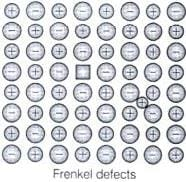(iv) Schottky defect is shown by ionic solids in which equal number of cation and anion get missed from ionic solids and thus, density of solid decreases.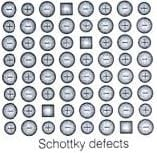Q.65. Match the type of unit cell given in Column I with the features given in Column II.

 Column I Column II (i) Primitive cubic unit cell (a) Each of the three perpendicular edges compulsorily have the different edge length i.e;  a≠b≠c. (ii) Body centred cubic unit cell (b) Number of atoms per unit cell is one. (iii) Face centred cubic unit cell (c) Each of the three perpendicular edges compulsorily have the same edge length i.e; a = b = c (iv) End centred orthorhombic unit cell (d) In addition to the contribution from the corner atoms the number of atoms present in a unit cell is one. (e) In addition to the contribution from the corner atoms the number of atoms present in a unit cell is three.

Ans. (i) → (b, c); (ii) → (c, d); (iii) → (c, e); (iv) → (a, d)
Solution.
(i) For primitive unit cell, a = b = c
Total number of atoms per unit cell = 1 / 8 x 8 = 1
Here, 1/8 is due to contribution of each atom present at corner.
(ii) For body centered cubic unit cell, a = b = c
This lattice contains atoms at corner as well as body centre. Contribution due to atoms at corner = 1 / 8 x 8 = 1 contribution due to atoms at body centre = 8
(iii) For face centred unit cell, a = b = c
Total constituent ions per unit cell present at corners = 1 / 8 x 8 = 1
Total constituent ions per unit cell present at face centre = 1 / 2 x 6 = 3
(iv) For end centered orthorhombic unit cell, a ≠ b ≠ c
Total contribution of atoms present at corner = 1 / 8 x 8 = 1
Total contribution of atoms present at end centre = 1 / 2 x 2 = 1
Hence, other than corner it contains total one atom per unit cell.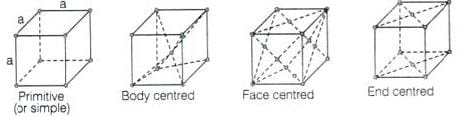Q.66. Match the types of defect given in Column I with the statement given in Column II.

 Column I Column II (i) Impurity defect (a) NaCl with anionic sites called F-centres (ii) Metal excess defect (b) FeO with Fe3+ (iii) Metal deficiency defect (c) NaCl with Sr2+ and some cationic sites vacant

Ans. (i) → (c); (ii) → (a); (iii)→ (b)
Solution.
(i) Impurity defects: The defects introduced in the crystal lattice due to presence of the certain impurity are called impurity defects.
Example: Substitution of Na+ ions in NaCl by Sr2+ ions.
Structure with defect: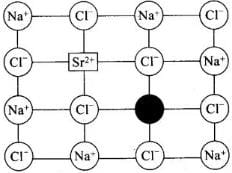Impurity defect due to substitution of Naions in NaCl by Sr2+ ions (Cation vacancy) ‘Schottky Defect’
(ii) When NaCl is heated in vapour of sodium some of the Cf leave their lattice site and create anion vacancies. This chloride ion wants to combine with sodium vapour to form sodium chloride. For doing so sodium atom loses electrons form Na+ ions. This released electron diffuses into the crystal to get entrapped in the anion vacancy called F-centre.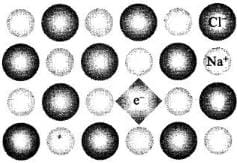(iii) Metal deficiency is caused due to cation vacancy created by replacement of some lower valent ions by its higher valentions.
Note: Cation vacancies are found in crystals in which metals have different oxidation states.
Example: FeO, FeS, NiO

Q.67. Match the items given in Column I with the items given in Column II.

 Column I Column II (i) Mg in solid state (a) p-Type semiconductor (ii) MgCl2 in molten state (b) n-Type semiconductor (iii) Silicon with phosphorus (c) Electrolytic conductors (iv) Germanium with boron (d) Electronic conductors

Ans. (i) → (d); (ii) → (c); (iii) → (b); (iv) →(a)
Solution.
(i) Mg in solid state show electronic conductivity due to presence of free electrons hence, they are known as electronic conductors.
(ii) MgCl2 in molten state show electrolytic conductivity due to presence of electrolytes in molten state.
(iii) Silicon doped with phosphorus contain one extra electron due to which it shows conductivity under the influence of electric field and known as p-type semiconductor.
(iv) Germanium doped with boron contains one hole due to which it shows conductivity under the influence of electric field and known as n-type semiconductor.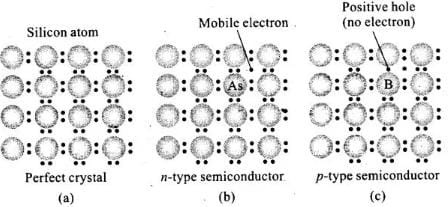Q.68. Match the type of packing given in Column I with the items given in Column II.

 Column I Column II (i) Square close packing in two dimensions (a) Triangular voids (ii) Hexagonal close packing in two dimensions (b) Pattern of spheres is repeated in every fourth layer (iii) Hexagonal close packing in three dimensions (c) Coordination number = 4 (iv) Cubic close packing in three dimensions (d) Pattern of sphere is repeated in alternate layers

Ans. (i) → (c); (ii) → (a); (iii) → (d); (iv) → (b)
Solution.
(i) Square close packing in two dimensions each sphere have coordination number 4, as shown below.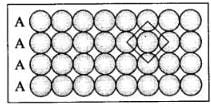(ii) Hexagonal close packing in two dimensions each sphere has coordination number 6 as shown below and creates a triangular void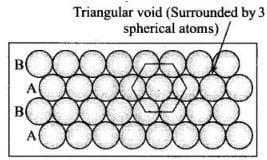(iii) Hexagonal close packing in 3 dimensions is a repeated pattern of sphere in alternate layers also known as ABAB pattern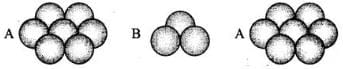(iv) Cubic close packing in a 3 dimensions is a repeating pattern of sphere in every fourth layer.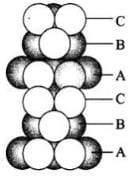ASSERTION AND REASON TYPE

Note : In the following questions a statement of assertion followed by a statement of reason is given.
Choose the correct answer out of the following choices.
(i) Assertion and reason both are correct statements and reason is correct explanation for assertion.
(ii) Assertion and reason both are correct statements but reason is not correct explanation for assertion.
(iii) Assertion is correct statement but reason is wrong statement.
(iv) Assertion is wrong statement but reason is correct statement.

Q.69. Assertion: The total number of atoms present in a simple cubic unit cell is one.
Reason: Simple cubic unit cell has atoms at its corners, each of which is shared between eight adjacent unit cells.
Ans. (i)
Solution.
In simple cubic unit cell, only comers are occupied by atoms. Thus, total number of atoms present in the unit cell will be one.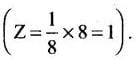Q.70. Assertion: Graphite is a good conductor of electricity however diamond belongs to the category of insulators.
Reason: Graphite is soft in nature on the other hand diamond is very hard and brittle.
Choose the correct answer out of the following choices.
(i) Assertion and reason both are correct statements and reason is correct explanation for assertion.
(ii) Assertion and reason both are correct statements but reason is not correct explanation for assertion.
(iii) Assertion is correct statement but reason is wrong statement.
(iv) Assertion is wrong statement but reason is correct statement.

Ans. (ii)
Solution.
Diamond is bad conductor of electricity because all valence of carbon are involved in bonding. In graphite however 3 out of 4 valence electrons are involved in bonding, fourth electron remains free between adjacent layers which makes it a good conductor.
Graphite is soft because parallel layers are held together by week van der Waals force. However, diamond is hard due to compact three-dimensional network of bonding.

Q.71. Assertion: Total number of octahedral voids present in unit cell of cubic close packing including the one that is present at the body centre, is four.
Reason: Besides the body centre there is one octahedral void present at the centre of each of the six faces of the unit cell and each of which is shared between two adjacent unit cells.
Choose the correct answer out of the following choices.
(i) Assertion and reason both are correct statements and reason is correct explanation for assertion.
(ii) Assertion and reason both are correct statements but reason is not correct explanation for assertion.
(iii) Assertion is correct statement but reason is wrong statement.
(iv) Assertion is wrong statement but reason is correct statement.

Ans. (iii)
Solution.
All edge centres and body centre represent octahedral void.
Total number of octahedral voids = 12 x 1/4 +1 = 4

Q.72. Assertion : The packing efficiency is maximum for the fcc structure.
Reason: The cordination number is 12 in fcc structures.
Choose the correct answer out of the following choices.
(i) Assertion and reason both are correct statements and reason is correct explanation for assertion.
(ii) Assertion and reason both are correct statements but reason is not correct explanation for assertion.
(iii) Assertion is correct statement but reason is wrong statement.
(iv) Assertion is wrong statement but reason is correct statement.

Ans. (ii)
Solution.
In fcc unit cell, there is cep arrangement with packing efficiency of 74.01% which is maximum. In cep arrangement, coordination number is 12.

Q.73. Assertion: Semiconductors are solids with conductivities in the intermediate range from 10–6 – 104 ohm–1m–1.
Reason: Intermediate conductivity in semiconductor is due to partially filled valence band.
Choose the correct answer out of the following choices.
(i) Assertion and reason both are correct statements and reason is correct explanation for assertion.
(ii) Assertion and reason both are correct statements but reason is not correct explanation for assertion.
(iii) Assertion is correct statement but reason is wrong statement.
(iv) Assertion is wrong statement but reason is correct statement.

Ans. (iii)
Solution.
Conductance of semiconductors lies between metals and insulators, i.e., in the range of 10-6 - 10ohm -1 m-1 .

LONG ASWER TYPE QUESTIONS

Q.74. With the help of a labelled diagram show that there are four octahedral voids per unit cell in a cubic close packed structure.
Ans. Cubic close packed structure contains one atom at each of eight corners of a unit cell and one atom at each of six faces which can be represented below:
As we know that any atom surrounded by six atoms (hard sphere) creates an octahedral void. In case of fcc body centre is surrounded by six identical atoms present at face centre hence, there is a octahedral void at body centre of each unit cell.Beside the body centre there is one octahedral void at centre of each of 12 edge as shown below: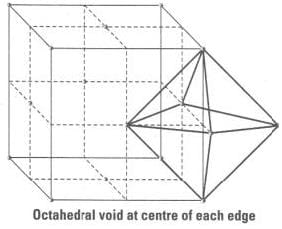Since, each void is shared by 4 unit cell. Therefore, contribution of octahedral void to each edge of a unit cell is 1/4.
Number of octahedral void at centre of 12 edge = 1 / 4 x 12 = 3
Number of octahedral void at body centre = 1
Therefore, total number of octahedral void at each ccp lattice = 3 + 1 = 4

Q.75. Show that in a cubic close packed structure, eight tetrahedral voids are present per unit cell.
Ans. Cubic close packed structure contains one atom at each corner of an unit cell and at face centre of each unit cell. Each unit cell consists of 8 small cubes.
Each small cube contains 4 atoms at its alternate corner when these atoms are joined to each other lead to creation of a tetrahedral void as shown below: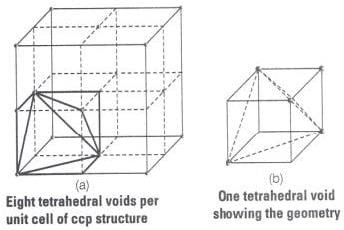Since, there are total 8 smaller cubes present at one unit cell and each smaller cube has one tetrahedral void hence, total number of tetrahedral void present in each unit cell is equal to eight.
As we know ccp structure has 4 atoms per unit cell. Thus, total number of tetrahedral void in one ccp unit cell is equal to 8.

Q.76. How does the doping increase the conductivity of semiconductors?
Ans. The conductivity of semiconductors is increased by adding an appropriate amount of suitable impurity or doping. Doping can be done with an impurity which is electron rich or electron deficient as compared to the intrinsic semiconductor, silicon or germanium. Such impurities introduce electronic defects in them. When silicon is doped with electron rich impurities the extra electron becomes delocalized. These delocalized electrons increase the conductivity of doped silicon due to the negatively charged electron, hence silicon doped with electron-rich impurity is called n-type semiconductor while electron-deficit impurities increase the conductivity through positive holes and this type of semiconductors are called /?-type semiconductors.

Q.77. A sample of ferrous oxide has actual formula Fe0.93O1.00. In this sample what fraction of metal ions are Fe2+ ions? What type of non-stoichiometric defect is present in this sample?
Ans. Let the formula of the sample be (Fe2+)x (Fe3+ )y O
On looking at the given formula of the compound
x + y = 0.93 …..(i)
Total positive charge on ferrous and ferric ions should balance the two units of negative charge on oxygen
Therefore, 2x + 3y = 2 ......(ii)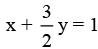.......(iii)
On subtracting equation (i) from equation (iii) we have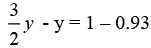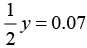⇒ y = 0.14
On putting the value of y in equation (i), we get
x + 0.14 = 0.93
⇒ x = 0.93 - 0.14
⇒ x = 0.79
Fraction of Fe2+ ions present in the sample =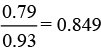Metal deficiency defect is present in the sample because iron is less in amount than that required for stoichiometric composition.

The document NCERT Exemplar: Solid State | Chemistry Class 12 - NEET is a part of the NEET Course Chemistry Class 12.
All you need of NEET at this link: NEET

## FAQs on NCERT Exemplar: Solid State - Chemistry Class 12 - NEET

 1. What is the definition of solid state in chemistry?Ans. Solid state in chemistry refers to the state of matter where particles are closely packed together in a fixed pattern and have a fixed shape and volume. It is one of the three states of matter, along with liquid and gas.
 2. What are the types of solid states?Ans. There are four main types of solid states: crystalline solids, amorphous solids, polymeric solids, and molecular solids. Crystalline solids have a regular and repeating arrangement of particles, amorphous solids have a disordered arrangement, polymeric solids are made up of long chains of repeating units, and molecular solids are held together by intermolecular forces.
 3. How are crystalline solids different from amorphous solids?Ans. Crystalline solids have a highly ordered and repeating arrangement of particles, while amorphous solids have a disordered and irregular arrangement. Crystalline solids have a definite melting point and distinct geometrical shapes, while amorphous solids do not have a specific melting point and can be molded into various shapes.
 4. What are the properties of solid state materials?Ans. Solid state materials have several properties such as high density, definite shape and volume, brittleness, high melting and boiling points, low compressibility, and strong intermolecular forces. They are also good conductors or insulators of heat and electricity, depending on their structure and composition.
 5. How are solid state materials classified?Ans. Solid state materials are classified based on their arrangement of particles and their properties. They can be classified as crystalline or amorphous based on their arrangement, and as metals, nonmetals, or metalloids based on their chemical nature. They can also be classified as conductors, semiconductors, or insulators based on their ability to conduct electricity.

## Chemistry Class 12

154 videos|396 docs|261 tests

## Chemistry Class 12

154 videos|396 docs|261 tests

### How to Prepare for NEET

Read our guide to prepare for NEET which is created by Toppers & the best Teachers
Signup to see your scores go up within 7 days! Learn & Practice with 1000+ FREE Notes, Videos & Tests.
10M+ students study on EduRev
Track your progress, build streaks, highlight & save important lessons and more!(Scan QR code)
Related Searches

,

,

,

,

,

,

,

,

,

,

,

,

,

,

,

,

,

,

,

,

,

;